# The Ξ function

This is the final article on fast-growing functions. In the first two articles we looked at computable functions, up to and including Friedman’s TREE function. The third article described the Busy Beaver function, which eventually overtakes all computable functions. This article will explore oracle Turing machines, Rayo’s number and ultimately give a inconceivably fast-growing function Ξ, such that Ξ(10^6) far exceeds any number anyone has yet defined.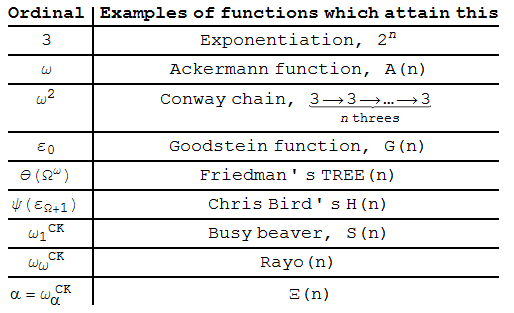### Oracle Turing machines

The first approach to creating functions faster than the Busy Beaver was to equip a Turing machine with a special ‘oracle’, which it can consult to solve the halting problem. These ‘second-order Turing machines’ are more powerful than ordinary Turing machines. The equivalent of the Busy Beaver function for second-order machines, S_2(n), cannot be expressed as any computable sequence of applications of the ordinary Busy Beaver function S. As such, it must correspond to the third admissible ordinal, ω2CK.

There is no need to stop there; we can define third-order Turing machines and so on. The kth-order Busy Beaver function S_k gives us the ordinal ωkCK.

If we can represent Turing machines of arbitrary order in a specific notation, it’s possible to define a function with growth rate corresponding to the ordinal ωωCK. The most elegant approach, considered by Rayo, was to use first-order logic as an alternative to Turing machines.

### First-order logic

In first-order logic, we have a set of symbols:

• Basic arithmetic operators, namely + and ×.
• Elementary natural numbers, 0 and 1.
• Parentheses, ( and ).
• Variables which can range over the natural numbers. We have an infinite alphabet of variables {a, b, c, d, …, z, a’, b’, c’, …, z’, a”, b”, …}, which can be reduced to a finite alphabet if we use ‘ (prime) as a separate symbol.
• The binary operator x == y, which gives a Boolean value (true or false) depending on whether the naturals x and y are equal.
• Basic logical operators, namely AND, OR and NOT. In principle, these can all be replaced with NAND.
• The existential quantifier ∃ and the universal quantifier ∀, together with ‘such that’ (represented by the colon, ‘:’).

For instance, Lagrange’s four square theorem can be written as ∀n:(∃a:(∃b:(∃c:(∃d:((a×a)+(b×b)+(c×c)+(d×d) == n))))). The busy beaver function can be expressed in first-order logic, as can any of the higher-order busy beaver functions mentioned above.

Rayo’s function Rayo(n) is defined as ‘the largest integer expressible uniquely by n symbols in first-order logic’. It must necessarily be ωωCK, since it can be bounded above by the diagonal sequence of nth-order Busy Beaver functions, but dominates any fixed-order Busy Beaver function. Rayo used his function to define a very large number, Rayo(10^100), to win a competition. We’re now going to use a third model of computation, combinatory logic, to absolutely annihilate this record.

### Combinatory logic

Combinatory logic uses the idea of combinators, which are functions mapping combinators to combinators. We usually use three primitive combinators, namely I (identity function), K and S. Applications of combinators can be represented as a binary tree, where the function is on the left and the argument is on the right. The ‘rules’ for applying I,K,S are displayed below: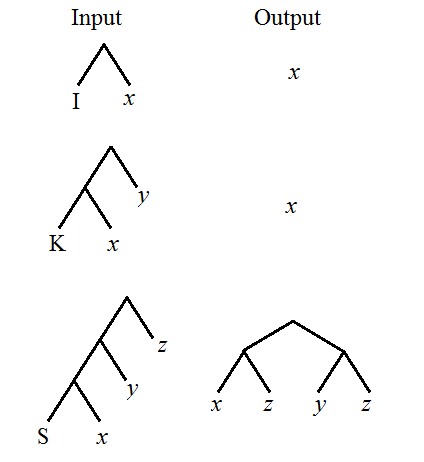The process of taking the leftmost combinator in a tree and applying the respective rule is known as beta reduction. Some things eventually β-reduce to give the identity combinator. An example of this is the following tree:Some trees do not reduce to the identity combinator. We say that this particular one has an output of size 2; this is the number of leaf nodes in the final stage of the β-reduction before it reaches a state where we can β-reduce no further.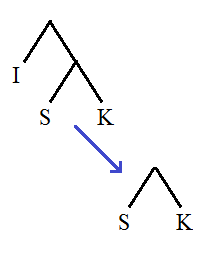And there are those which grow infinitely: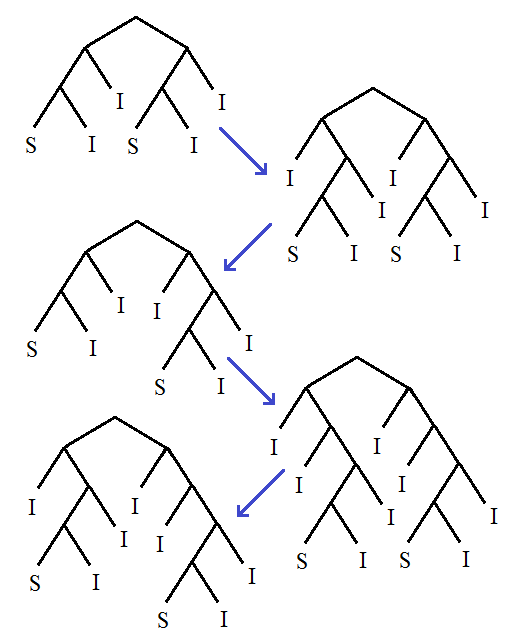With these three combinators, it’s possible to emulate Turing machines. We can represent natural numbers with functions known as Church numerals and perform arithmetic and logical operations. Recursion and iteration are also possible in this system.

### Generalised combinatory logic and the Ξ function

Trees of SKI combinators are no more powerful than Turing machines. Nevertheless, we can actually strengthen it considerably by introducing a new super-combinator, the oracle combinator Ω. This accepts three arguments, and returns the second argument if and only if the first argument β-reduces to I, and returns the third argument otherwise. Here is a visual depiction: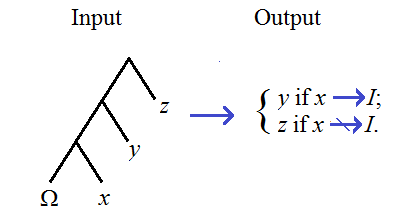It’s possible to define paradoxical, nonsensical, self-referential statements. We’ll remove this contradiction by being careful and only concentrating on well-founded trees. Firstly, trees consisting entirely of S, K and I are called ‘rank 0’. If, in the sequence of beta-reductions of a tree T, we only have to apply Ω to trees of rank less than the ordinal α, then we say that T has rank α. Trees with ranks are known as well-founded, as the definition resembles that of a set obeying the axiom of foundation (and thereby inhabiting the von Neumann universe).

We can build a rank-2 tree capable of computing the Busy Beaver function. We can also build existential and universal quantifiers using the oracle combinator, so a n-quantified expression in first-order logic can be evaluated by a rank-(n+1) tree. Hence, using a rank-ω tree, we can compute Rayo’s function. It shouldn’t be too difficult to imagine this requiring far fewer than 10^6 combinators.

I define my function, Ξ(n) to give the size of the largest output of a well-founded tree of n combinators. Consequently, Ξ(10^6) is inconceivably larger than the largest number defined before, namely Rayo(10^100). If you look at the ordinal table at the top of the page, you’ll see how much faster Ξ is than Rayo’s function.

### Beyond Ξ?

If you add another oracle combinator, Ω’, capable of testing whether an expression is well-founded, then we can compute the Ξ function and much more. The variant of Ξ defined for the combinatory logic defined using {S, K, I, Ω, Ω’} is called Ξ_2, and has an even faster growth rate. You can continue adding further oracle symbols to obtain even faster-growing functions. I’m not going to bother doing this, though, since it’s really quite pointless as we have already broken the record.

This entry was posted in Fast-growing functions. Bookmark the permalink.

### 50 Responses to The Ξ function

1.wojowu says:

I don’t really understand one thing – SKI (and its generalization too, I guess) concerns finite trees only, so they can’t be self referential without equipping ourselves with infinite branch containing itself over and over. If this is a case, why can’t we do that on standard SKI?
Also, did you came with oracle operator yourself/with friends, or is it some better known concept? I wonder if there are any publications about that.

•apgoucher says:

Finite trees can be self-referential in ordinary SKI. There’s a combinator, Y = S (K (S I I)) (S (S (K S) K) (K (S I I))), which has the property that Y(x) beta-reduces to x Y(x).

I devised the oracle operator to specify nth-order Turing machines in a canonical form. The concept of an oracle Turing machine was created much earlier (by Turing himself, I believe) and is well documented.

•Thomas says:

Actually, there is a shorter combinator that does this: SI(S(K(SI)))(SII)

•Thomas says:

Also, the shortest paradoxical combinator that ‘i could find is SI(S(K(SI)))(SII)(S(SO(KK))(KI))

2.Anonymous says:

What are the known values of the xi function?

•Thomas says:

The known values are:
Xi(1)=1
Xi(2)=2
Xi(3)=3
Xi(4)=4
Xi(5)=6
Xi(6)=17

•Thomas says:

Oh, and the combinators for those are S,SS,SSS,SSSS,SSS(SI),SSS(SI)S. Also, Xi(7)>=40 (and the combinator for that is SS(SI)(SS))

•apgoucher says:

SS(SI)(SS)) is neither parenthetically balanced nor has 7 combinators…?

•Thomas says:

woops, I meant SSS(SI)(SS)

•apgoucher says:

Thanks.

•Thomas says:

Actually, I just found that Xi(7)>=51, and the combinator for that is: SSS(SI)S\(\Omega\)

•Thomas says:

SSS(SI)SO, where O is the oracle combinator

•apgoucher says:

I’ve verified each of your combinators with this Mathematica routine I wrote:

p[s[s][s][s[i]][s[s]]] //. {p[x_[y_]] -> p[x][y],
p[s][x_][y_][z_] -> p[x[z][y[z]]], p[k][x_][y_] -> p[x],
p[i][x_] -> p[x]}

The input is on the left of //. and the pattern-processing rule is on the right. The p function is used to ensure that only the leftmost combinator is processed.

Replacing //. with /. causes it to perform a single step. I suppose I could modify this program to check for periodicity etc.

•Thomas says:

Including SSS(SI)SO?

•apgoucher says:

I did the applications of O manually, since it can’t be done algorithmically (except in special cases, such as when the first argument becomes irreducible or periodic).

•Thomas says:

I’ve almost finished searching through the possibilities for 7 combinators. I just have to finish searching through the type: Suv(wx)yz, and search through Suv(wx)(yz). I’ll post back in an hour when my analysis is complete.

•Thomas says:

I have completed my analysis, and no other (length 7) combinator grows to over 51 combinators long.

•Thomas says:

I was wondering if you could try to beta-reduce the combinator: SSS(SI)(SS)O. It has multiple applications of the O combinator, some inside others

•Thomas says:

Actually, I solved this, it diverges eventually. What I’m having trouble with now is SSS(SI)S(S(S(S(S(SO))))). I got to 948 nested applications of O before python hit the max recursion depth.

•Thomas says:

In the article you talk about the rank of a combinator expression. Then you talk about combinators with rank w. Could you give an example of an expression of rank w?

3.Yura says:

Very intresting. thank you.

4.wojowu says:

In “Oracle Turing machines” section you said that S_2(n) can’t be expressed as computable sequence of aplications of S(n). But I don’t really understand why it implies that it corresponds to ω1CK × 2. I agree that this is obvious lower bound, but you didn’t prove that it’s also upper bound. Using S(n) we can solve halting problem, and thus we can use it to represent ω1CK, so (if I’m right) we can “use” that ordinal and diagonalize through it. In this way, we can create function corresponding to ω1CK × 2 computable using S(n) oracle, so strictly weaker than S_2(n). If this is true, my guess for strength of the function is ω2CK, third admissible ordinal.

•apgoucher says:

Oh, yes, my deductions were erroneous, so you’re probably correct. How is ω2CK defined? I can’t find any published papers on this subject.

•wojowu says:

ω2CK is exactly what I said – third admissible ordinal. Admissible ordinals are defined using Godel constructive hierarchy of sets. If L_α is admissible set (i.e. model of Kripke–Platek set theory), then α is admissible ordinal. ω and ω1CK are first two such ordinals.
According to this paper http://www.math.uni-bonn.de/people/koepke/Preprints/Ordinal_machines_and_admissible_recursion_theory.pdf admissible ordinals are ω1CK analogues for oracle machines.
Also, you should revise ω element in Rayo(n) function. I’m pretty sure whole first-order logic is able to create ordinal notations, and don’t forget that oracle machines are not limited to finite degrees. You can diagonalize through them as well. Good luck with ordinal analysis 😉

•apgoucher says:

Rayo(n) is certainly ωωCK, since I can lower-bound it by all ordinals ωnCK (any nth-order Turing machine can be expressed in first-order logic) and upper-bound it with ωωCK (every statement with n quantifiers in normal form can be tested by a nth-order oracle Turing machine). I believe that the Ξ function corresponds to the first fixed point of α –> ωαCK.

•wojowu says:

Take halting problem oracle for every order oracle machine. Then apply Cantor pairing function to them. It is computable, as well as its inverse, so Turing machine with such oracle can encode it and solve halting problem for every finite order. So it is order ω oracle machine. Isn’t such machine expressable in first-order logic?
Well, computably large oracle trees certainly can compute ωαCK given α, so every ωαCK lower than your fixed point is strictly weaker than Ξ function, but still – this is only lower bound.

•apgoucher says:

The order-omega halting problem cannot be expressed in first-order logic, since every order of oracle requires an extra quantifier.

•Ikosarakt says:

Put the definition of ω(a+1)CK simpler: suppose that we can compute ωaCK[n] and then we can apply any computable function to ωaCK and any smaller ordinal. Then ω(a+1)CK is the first ordinal which can’t be expressed in these terms.
Thus, ω2CK must be larger than ω1CK*2, ω^(ω1CK+1), epsilon_(ω1CK+1), zeta_(ω1CK+1), gamma_(ω1CK+1), and generally alpha_(ω1CK+1), where alpha_0 is a recursive ordinal.

•wojowu says:

That’s right, expect for alpha_0. If alpha_0 is recursive, we have no guarantee that alpha_1 will be recursive. Simple example for that is that ω can be considered to be ω^CK_0, and ω^CK_3 is already greater than ω2CK.

5.Deedlit says:

I wrote the following in the Googology Wiki concerning your evaluation of Rayo’s number: (I hope you don’t mind me copying and pasting rather than writing it all over again.)

Apparently, in a blog post a few months ago, A.P. Goucher made the claim that Rayo’s number was at the level of the order-ω Busy Beaver function (and there at the level of ωCK_ω in the fast-growing hierarchy, although this association has issues of its own). He therefore concluded that his Xi function grew faster. These statements have been repeated several times in this wiki. However, it is incorrect.

The trouble is that Goucher got the definition of Rayo’s number wrong. The correct definition is, per the wiki article, “the smallest number bigger than any finite number named by an expression in the language of first order set theory with a googol symbols or less.” Goucher, however, defined it as “the largest integer expressible uniquely by n symbols in first-order logic”. He then goes on to define first-order logic, but what he is actually defining is first-order arithmetic.

Now, the “largest integer expressible uniquely by n symbols in first-order arithmetic” is indeed on the order of the order-ω Busy Beaver function. But second order arithmetic is much, much stronger; it is measured by Turing hyperjumps, which are on a completely different level from the Turing jumps of first-order arithmetic. So I don’t think we are even equipped to measure the strength of second-order arithmetic in terms of higher order Busy Beaver functions. And first order set theory is much, much stronger than second-order arithmetic, or even nth-order arithmetic for any n. I’m not sure how strong Goucher’s combinatory logic is, but it doesn’t seem to have the power of second order arithmetic. So I strongly believe that Rayo’s function grows much, much faster than Goucher’s Xi function.

What do you think?

6.apgoucher says:

How are you defining ‘first-order set theory’? Specifically, what is the domain of discourse over which you’re quantifying? Since it was unspecified, I assumed the integers, in which case it’s equivalent to Peano arithmetic. I admit that if you’re quantifying over a different domain of discourse, then it could quite possibly be more powerful.

•Deedlit says:

“First order logic” is the one in which the domain of discourse is unspecified. In first order set theory, the domain of discourse is defined to be sets. I’m sure this is what Rayo intended; if he had wanted the domain of discourse to be natural numbers, he would have said first order arithmetic rather than first order set theory. I’ll bet first order arithmetic was used for an earlier number in the Big Numbe Duel.

•apgoucher says:

Sets of what, exactly? The entire von Neumann universe?

•Deedlit says:

Yes indeed.

•apgoucher says:

In that case, Rayo’s function is almost certainly more powerful than the Xi function. However, is it not possible to implement the following ideas as statements in first-order set theory?

1. “A is a subset of B” = for all a in A, there exists b in B such that a = b.

2. “A is the powerset of B” = for all C, C is in A if and only if C is a subset of B.

3. “A is injectable into B” = there exists a set C such that (for all a in A, there exists c in C and b in B such that a is in c and b is in c) and (there do not exist d, e in C such that there exists b in B such that b is an element of both d and e, but d is not equal to e).

4. “C is the set of pairs of elements of A” = (C is a subset of the powerset of A) and (for all a,b in A, there exists c in C such that a and b are elements of C) and (for all c in C, there do not exist x, y, z in C such that x,y,z are distinct) and (for all c in C, there exist x, y in C such that x,y are distinct).

5. “A is infinite” = the set of pairs of elements of A is injectable into A, and A contains at least four distinct elements.

6. “A has the same cardinality as the naturals” = for every infinite set X, A is injectable into X.

7. “The continuum hypothesis is true” = there exists a set A with the same cardinality of the naturals, such that there exists a set B which is the powerset of set A, such that there exists a set C which is a subset of set B, and such that (B is not injectable into C, and C is not injectable into A).

In that case, do you define the statement corresponding to 7 to be true or false? It’s independent of ZFC.

(It’s entirely possible that I made a mistake somewhere along the way, as this feels worryingly powerful for first-order logic.)

•Deedlit says:

You are quite right! The continuum hypothesis is definable in the language of set theory. So Rayo is assuming that there are statements independent of ZFC, or any sound formal theory of sets, that have a definite truth value. Note that this this is necessary for the growth rate of Rayo’s function; if we restrict the notion of truth to provable or disprovable statements only, then we could have a Turing machine search through all possible proofs of ZFC until it found a proof or disproof of a statement, so it turns out that Rayo’s function is dominated by the order-2 Busy Beaver function. Details can be found here:

http://mathoverflow.net/questions/34710/succinctly-naming-big-numbers-zfc-versus-busy-beaver

The same is true for any recursive theory. Even the Busy Beaver runs into this problem; for any recursive theory T, T decides only finitely many values of the Busy Beaver function. All higher values are independent on T. So to even go beyond the computable functions, we need some notion of truth beyond what is provable or disprovable.

Note that it is not necessary for ALL statements to have a truth value; it could be that some independent statements have a definitive truth value, and some do not. One may decide that the continuum hypothesis has no truth value, but a sufficient collection of statements do so that Rayo’s function far exceeds the similar function defined for second order arithemetic for example.

Now, one might argue that, in universes with finitary objects, like first order arithmetic and your combinatory logic, it is more plausible that every statement has a truth value. I agree with that. But it is a sliding scale; everyone will have a different opinion of just how far you can go before you the notion of truth dissipates.

7.jiawei says:

I think that this could be the winner unlike Rayo(n).

8.quelst says:

I have a question about moving beyond Ξ_2. You said that one could do this by introducing additional oracle operators beyond Ω’, but I am having trouble seeing how to do this.

The first oracle combinator Ω, as I understand it, is there to solve the halting problem which makes Busy Beavers uncomputable. Adding it to the SKI calculus lets us nest the oracles recursively, such that a rank-n tree can compute Busy Beavers for a rank-(n-1) tree. Ξ gets its power from the fact that the maximum rank for the tree which gives the output for Ξ(N) is limited only by the magnitude of N.

You point out that although this basically takes care of the halting problem, there are still trees which are not well-founded, and Ξ must ignore those, just as the Busy Beaver function must ignore non-halting trees. Here we bring in Ω’ which checks if an expression is well-founded; adding this to the combinator list gives Ξ_2, thus allowing us to compute the Ξ function and beyond.

My question is this: how do we move into Ξ_3 (and then on to Ξ_n of course)?
The Ω combinator takes care of halting problems, the Ω’ checks well-foundedness, but what does the next tier oracle, Ω’’, check? There must be trees in {S, K, I, Ω, Ω’} that are so badly behaved that even though you can check for halting, and for well-foundedness, they can still create problems only solvable by Ω’’, right?

•Thomas says:

There are some commutators that give a contradiction even when asked if given a contradiction. If P goes to O’PP’P”, where P” is well founded but P’ is not, then it gives a “double contradiction”, which is checkable with O”

•quelst says:

Ah, okay, that makes sense. The idea of Nth degree contradiction is fascinating… I will have to think that over – it isn’t hard to imagine that it continues working to the limit of Ξ_N. I wonder what the next meaningful step beyond that would be… anyway, thanks for clearing that up.

9.Anonymous says:

Has anyone found Xi(8) yet?

10.hypcos says:

What you introduced is not “SKI combinatory logic with oracle combinator”. Your definition of “SKI combinatory logic” and “beta-reduction” were wrong, or you have defined different things.

In SKI combinatory logic, everywhere in the expression should be reduced by the rules, not only the leftmost terms. For example, in your definition, SS(KS)I -> SI(KSI), then it can’t reduce any further; but in The SKI combinatory logic, the (KSI), which is not at the leftmost position, can further reduce to S, so SS(KS)I -> SI(KSI) -> SIS.

I doubt your definition of “SKI combinatory logic”, or even your Ξ function, is weaker than The SKI combinatory logic, since some reducable terms in The SKI combinatory logic don’t reduce in your definition.

11.Anonymous says:

Xi function does not grow faster than Rayo’s function. By definition, Rayo’s function grows faster than any function that can be expressed in first-order set theory in n symbols or less. Xi of 1 million, can be proven to be expressable in a million symbols.

12.AMOGUS says:

I know that I’m really late to this, but since Rayo’s Rayo(n) function is defined as (and I’m paraphrasing) “The smallest number bigger than any finite number that can be expressed in the language of first-order set theory with n symbols or less” I can guarantee it to be FAR bigger than this function you have defined. Because first-order set theory can define functions themselves, Rayo’s function diagonalizes through basically all definable functions that don’t use some extension of Rayo’s function itself. This means that your Xi function will eventually be defined (possibly very quickly), and will easily be outgrown by the Rayo function. Even trying to reach Rayo’s number (Rayo(10^100)) would be practically impossible using basically ANY form or extension of the Xi function, and that is NOTHING compared to actually creating a function that grows faster than Rayo(n).

In simple terms, your function that you claimed was going to “absolutely annihilate this record” doesn’t even come close.

13.Tor O. Idalet says:

Unfortunately, you made one mistake. Your reasoning appears to be as follows:
1. Because first-order set theory can define functions themselves, Rayo’s function diagonalizes through basically all definable functions that don’t use some extension of Rayo’s function itself.
2. Therefore, the Xi function will eventually be defined, and will easily be outgrown by the Rayo function.

There are two missing steps here. The first is:
0.5. Any function that does not use some extension of Rayo’s function itself is (or eventually becomes) smaller than Rayo’s function.
Lest you think I am putting words into your mouth, observe the following. If there is a function that “doesn’t use some extension of Rayo’s function” and is larger than Rayo(n), statement 1 falls apart. Therefore, statement 1 implies statement 0.5. Do you agree?

Here is the second missing step:
1.5. The Xi function does not use some extension than Rayo’s function itself.
Again, let me show you why wee need this. If you assumed that the Xi function did, in fact, use some extension of Rayo’s function itself, statement 2 would fall flat on its face. Do you agree that statement 1.5 is also necessary?

Now, from 0.5, we know that any function that does not use some extension of Rayo’s function itself is (or eventually becomes) smaller than Rayo’s function. We can therefore replace all instances of “does not use some extension of Rayo’s function itself” with “is smaller (eventually) than Rayo’s function itself”. The rest of the proof becomes as follows:
1. Because first order set theory can define functions themselves, Rayo’s function diagonalizes through basically all definable functions that are or eventually become smaller than Rayo’s function itself.
1.5. The Xi function is or eventually becomes smaller than Rayo’s function itself.
2. This means that the Xi function will eventually be defined, and will easily be outgrown by the Rayo function.

In other words, the Xi function is or eventually becomes smaller than the Rayo function therefore the Xi function will easily be outgrown by the Rayo function.
Now, this is a true statement (see https://cp4space.hatsya.com/2013/01/06/fast-growing-4/#comment-1073 and comments above it), but you did not prove it.

The missing steps might have seemed too simple to mention. But, in this case, they were not.

14.Tor O. Idalet says:

I was trying to reply to AMOGUS. Maybe the imposter is to blame?

•AMOGUS says:

I am new to this whole googology topic so I am sorry if I forgot the proof. I was just trying to give a very basic idea of my reasoning about his claims.

I will make sure to analyze my statements for detailed proof from now on. Thank you for pointing out this mistake.

•AMOGUS says:

Also note that I was assuming that a reader of this comment would have understood that since Rayo’s number could diagonalize through all functions that didn’t use some extension of Rayo’s function, that it would have been clear that it could have used that function (or a faster one that had been defined) to create a larger number of the same input n for the given function and Rayo’s function. I was also going on the assumption that a reader would have been able to compute the definition of the xi function and understand that it didn’t use some sort of extension of first-order set theory.

15.Tor O. Idalet says:

Thanks for replying! You can tell I wrote the post expecting a more hostile response, and it’s nice to know you weren’t that type of person. I think I might have been a little more dismissive than I should have been, and “someone is wrong on the internet” is not a good reason to write a reply.
It seems we just thought that different things were “obvious” (“Just as all the students were leaving, the professor returned, said ‘Yes, it is obvious,’ and continued the lecture”). After thinking about it for a bit, I realized it’s actually quite easy to define the function in first-order set theory (it comes straight out of the definition: define well-orderedness as in the post, represent a tree by its whole ssequence of beta-reductions and assert this is a finite sequence).